# How to Make a Simple Histogram with Altair in Python?

• Last Updated : 03 Jan, 2021

Prerequisites: Altair

Simple Histogram is the representation of frequency distribution by means of rectangles whose width represents the class interval. Histogram is the graphical representation that organizes grouped data points into the specified range. By using histogram we can visualize large amount of data and it’s frequency as one contiguous plot.

Attention geek! Strengthen your foundations with the Python Programming Foundation Course and learn the basics.

To begin with, your interview preparations Enhance your Data Structures concepts with the Python DS Course. And to begin with your Machine Learning Journey, join the Machine Learning - Basic Level Course

Installation:

For installing the altair library and vega_datasets we have the run the following command in our command prompt.

```pip install altair
pip install vega-datasets```

In this article we are going to plot Simple histogram with the help of altair library by using cars and iris datasets in the below various examples.

Step-by-step Approach:

• Import the library.
• Create or load the datasets.
• Select the column from the dataset on which we want to make the histogram.
• For making histogram, in altair library we have to give three important elements(altair.Chart(), mark_bar(), encode()).
• Put it in the variable let be named as “hist”.
• Then to see the plot we have to write the one line of code which is hist.show() and we are done.

Syntax:

altair.Chart(name_of_dataset).mark_bar().encode(x =”name_of_col1″, y=”name_of_col2″)

Example 1: Printing the dataset of cars()

## Python

 `# importing libraries``import` `altair as alt``from` `vega_datasets ``import` `data``import` `vega_datasets`` ` `# importing cars dataset form``# vega_datasets provided by altair``car_data  ``=` `data.cars()`` ` `# printing the dataset``display(car_data)`

Output: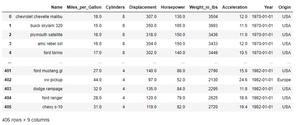Example 2: Making the default Simple Histogram using the cars dataset.

## Python

 `# importing libraries``import` `altair as alt``from` `vega_datasets ``import` `data`` ` `# importing cars dataset``# form vega_datasets provided by altair``car_data  ``=` `data.cars()`` ` `# making the simple histogram on Acceleration``hist ``=` `alt.Chart(car_data).mark_bar().encode(x ``=` `'Acceleration'``,``                                             ``y ``=` `'count()'``)``# showing the histogram``hist.show()`

Output: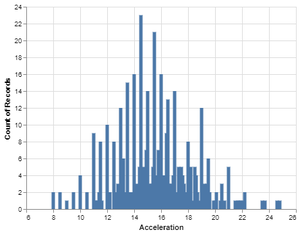Example 3: Making the Simple Histogram using the cars dataset by setting the bin on Acceleration.

## Python

 `# importing libraries``import` `altair as alt``from` `vega_datasets ``import` `data`` ` `# importing cars dataset``# form vega_datasets provided by altair``car_data  ``=` `data.cars()`` ` `# making the simple histogram``# on Acceleration by setting the bin``hist ``=` `alt.Chart(car_data).mark_bar().encode(x ``=` `alt.X(``'Acceleration'``,``                                                       ``bin` `=` `alt.BinParams(maxbins ``=` `30``)),``                                             ``y ``=` `'count()'``)``# showing the histogram``hist.show()`

Output: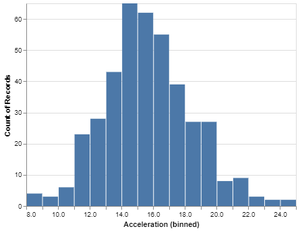Example 4: Making the Simple Histogram using the cars dataset by setting the bin on Horsepower.

## Python

 `# importing libraries``import` `altair as alt``from` `vega_datasets ``import` `data`` ` `# importing cars dataset``# form vega_datasets provided by altair``car_data  ``=` `data.cars()`` ` `# making the simple histogram``# on Horsepower by setting the bin``hist ``=` `alt.Chart(car_data).mark_bar().encode(x ``=` `alt.X(``'Horsepower'``,``                                                       ``bin` `=` `alt.BinParams(maxbins ``=` `20``)),``                                             ``y ``=` `'count()'``)``# showing the histogram``hist.show()`

Output: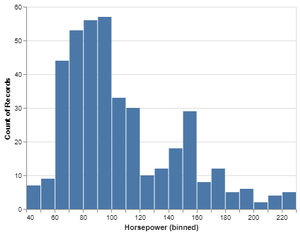Example 5: Making the default Simple Histogram using iris dataset on sepalLength.

## Python

 `# importing libraries``import` `altair as alt``from` `vega_datasets ``import` `data`` ` `# importing cars dataset``# form vega_datasets provided by altair``iris_data  ``=` `data.iris()`` ` `# making the simple histogram on sepal length``hist ``=` `alt.Chart(iris_data).mark_bar().encode(x ``=` `'sepalLength'``,``                                              ``y ``=` `'count()'``)`` ` `# showing the histogram``hist.show()`

Output: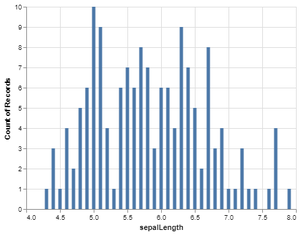We can also print the iris dataset using print(iris_data) in the program after loading the iris dataset.  Our iris dataset after printing it looks like this.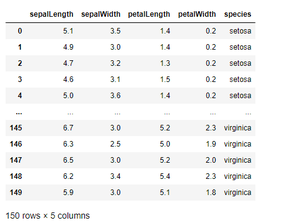Example 6: Making the Simple Histogram by setting bin and color.

## Python

 `# importing libraries``import` `altair as alt``from` `vega_datasets ``import` `data`` ` `# importing cars dataset``# form vega_datasets provided by altair``iris_data  ``=` `data.iris()`` ` `# making the simple histogram``# on sepal length by setting bin``# and color on the basis of species``hist ``=` `alt.Chart(iris_data).mark_bar().encode(x ``=` `alt.X(``'sepalLength'``,``                                                        ``bin` `=` `alt.BinParams(maxbins ``=` `20``)),``                                              ``y ``=` `'count()'``,color ``=` `'species'``)``# showing the histogram``hist.show()`

Output: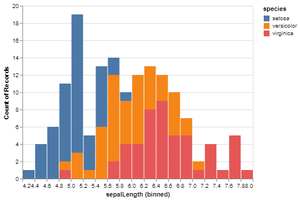In the same way we can make the simple histogram on any values of dataset and can set the color accordingly.

Example 7: Making Simple Histogram using iris dataset on petal width by setting bin and color.

## Python

 `# importing libraries``import` `altair as alt``from` `vega_datasets ``import` `data`` ` `# importing cars dataset``# form vega_datasets provided by altair``iris_data  ``=` `data.iris()`` ` `# making the simple histogram``# on petal width by setting bin``# and color on the basis of species``hist ``=` `alt.Chart(iris_data).mark_bar().encode(x ``=` `alt.X(``'petalWidth'``,``                                                        ``bin` `=` `alt.BinParams(maxbins ``=` `10``)),``                                              ``y ``=` `'count()'``, color ``=` `'species'``)``# showing the histogram``hist.show()`

Output: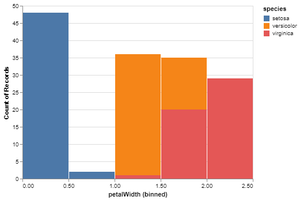My Personal Notes arrow_drop_up